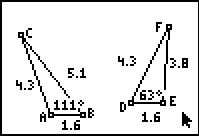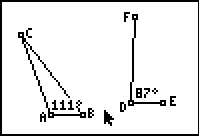# Activities

••• ##### Subject Area

• Math: Geometry: Triangles

• ##### Author9-12

40 Minutes

• ##### Device
• TI-83 Plus Family
• TI-84 Plus
• TI-84 Plus Silver Edition
•TI-84 Plus C Silver Edition
•TI-84 Plus CE
• ##### Software

TI Connect™
TI Connect™ CE

• ##### Report an Issue

The Hinge Theorems#### Activity Overview

Students explore the inequality relationships that arise when some of the triangle congruence conditions are in place but others are not.

#### Key Steps

•Students will construct a triangle and then use the Compass tool to construct segments that are congruent to the original triangle. Using the two triangles constructed students will investigate the SAS Inequality Theorem. By dragging point F, they will be able to see how the lengths of the sides differ when angle D is greater than or less than angle A.

•In the second problem, students will use the same setup of the two triangles to investigate the SSS Inequality Theorem. By dragging point E or point F they will be able to see how the measures of angle D and angle A compare when segment EF is longer than or shorter than segment BC.